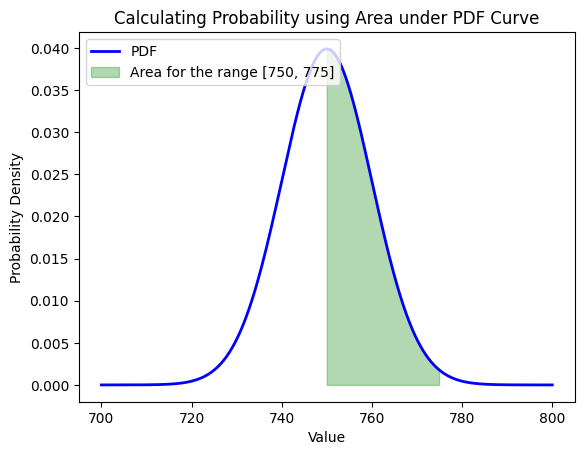Course Content

Probability Theory Mastering

##Cumulative Distribution Functions and Probability Density Functions

The Cumulative Distribution Function (CDF) is a function that describes the cumulative probability of a random variable taking on a value less than or equal to a given value.

Mathematically, the CDF of a random variable X, denoted as F(x), is defined as:

F(x) = Probability that variable X is less or equal to value x.

Using this function, it is easy to describe continuous random variables. Look at the example below: we will use a normally distributed random variable (Normal distribution was considered in the Probability Theory course) and look at its CDF.Using CDF, we can determine the probability that our random variable belongs to any of the intervals of interest. Assume that X is a random variable, and F(x) is its CDF.
To determine the probability that the variable X belongs to the interval [a, b], we can use the following formula:

P{X є [a,b]} = F(b) - F(a).We can also use CDF with discrete random variables and calculate probabilities using the formula which was described above:Percent Point Function (PPF), also known as the inverse of the cumulative distribution function (CDF). It is used to find the value of a random variable that corresponds to a given probability. In Python it is implemented using `.ppf()` method:Probability Density Function (PDF) is a function that provides information about the likelihood of a random variable taking on a particular value at a specific point in the continuous range. The PDF defines the shape of the probability distribution of a continuous random variable. Let's look at the example:The PDF can be interpreted as relative likelihood or probability density. Higher values of the PDF at a particular point in the continuous range indicate that the random variable is more likely to take on that value. Conversely, lower values of the PDF at a particular point in the range indicate that the random variable is less likely to take on that value. Thus we can conclude that we can't use PDF directly to determine probabilities, but it is used to determine various properties and characteristics of a random variable.

Mathematically, PDF is the derivative of the CDF: we can calculate the probability that a continuous random variable belongs to a certain interval as the area of the figure bounded by the PDF plot from above and by the limits of the corresponding interval from the sides.#Choose the correct statements about the calculation of the probability random variables belong to a certain interval

## Select a few correct answers

Everything was clear?

Section 1. Chapter 2